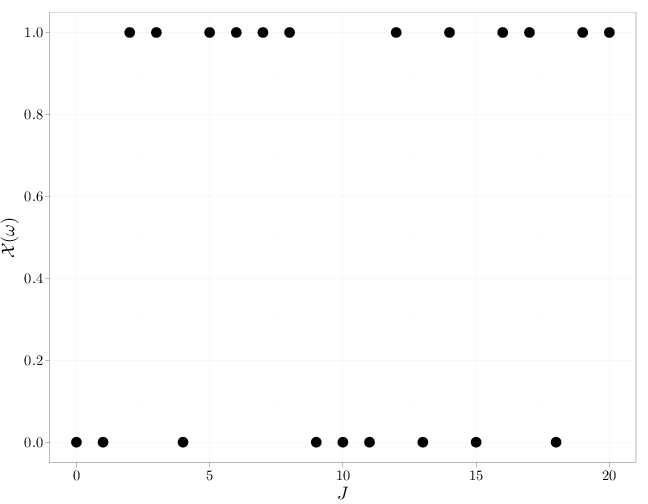## Probability

### The Analysis of Data, volume 1

Random Processes: Random Processes and Marginal Distributions

## 6.2. Random Processes and Marginal Distributions

Given a random process we can consider its finite dimensional marginals, namely the distribution of $X_{t_1},\ldots,X_{t_k}$ for some $k\in\mathbb{N}$ and some $t_1,\ldots,t_k\in J$. The marginals distributions are characterized by the corresponding joint cdf functions $F_{X_{t_1},\ldots,X_{t_k}}(r_1,\ldots,r_k) = \P(X_{t_1}\leq r_1,\ldots,X_{t_k}\leq r_k)$ where $k\geq 1$, $t_1,\ldots,t_k\in J$ and $r_1,\ldots,r_k\in\R$. If the process RVs are discrete, we can consider instead the joint pmf functions, and if the process RVs are continuous, we can consider instead the joint pdf functions.

Example 6.2.1. Consider a random process similar to the one in Example 6.1.1, with $J=\R$ and $X_t=Y\cos(2\pi t)$ for some discrete RV $Y$ with pmf $p_Y$. Since each $X_t$ is a function of any other $X_{t'}$ \begin{align*} p_{X_{t_1},\ldots,X_{t_k}}(r_1,\ldots,r_k)= \begin{cases} p_Y(r_j/\cos(2\pi t_j) & r_j/\cos(2\pi t_j)=r_i/\cos(2\pi t_i) \,\,\forall i,j\\ 0 & \text{otherwise} \end{cases}. \end{align*}

Given an RP $\mathcal{X}=\{X_t:t\in J\}$, all finite dimensional marginals are defined. Kolmogorov's extension theorem below states that the reverse also holds: a collection of finite dimensional marginal distributions uniquely defines a random process, as long as the marginals do not contradict each other. Consequentially, we now have a systematic way to define random processes by defining a collection of all finite dimensional marginals (that are consistent with each other).

Definition 6.2.1. A collection of finite dimensional marginal distributions $\mathcal{L}$ are said to be consistent if the following two conditions apply:
1. If $F_{X_{t_1},\ldots,X_{t_k}}\in\mathcal{L}$ and $F_{X_{t_1},\ldots,X_{t_k},X_{t_{k+1}}}\in\mathcal{L}$ then for all $r_1,\ldots,r_{k+1}\in\R$ \begin{align*} F_{X_{t_1},\ldots,X_{t_k}}(r_1,\ldots,r_k) = \lim_{r_{k+1}\to+\infty} F_{X_{t_1},\ldots,X_{t_k},X_{t_{k+1}}}(r_1, \ldots,r_k, r_{k+1}). \end{align*}
2. If $\mathcal{L}$ has two finite dimensional marginal cdfs over the same RVs, the order in which the RVs appear is immaterial; for example, $F_{X_{t_1},X_{t_2}}(r_1,r_2)=F_{X_{t_2},X_{t_1}}(r_2,r_1).$

The finite dimensional marginals arising from a random process satisfy the consistency definition above since they are derived from the same joint distribution $\P$ over the common sample space $\Omega$. Kolmogorov's extension theorem below states that the converse also holds. The proof for discrete time RPs is available in Section 6.5. A proof for continuous time RPs is available in (Billingsley, 1995).

Proposition 6.2.1 (Kolmogorov's Extension Theorem). Given a collection $\mathcal{L}$ of all possible finite dimensional marginal cdfs that is consistent in the sense of Definition 6.2.1, there exists a unique random process whose finite dimensional marginals coincide with $\mathcal{L}$.

Instead of specifying a process by its finite dimensional marginal cdfs, we may do so using all finite dimensional marginal pdfs (if $X_t$, $t\in J$ are continuous) or all finite dimensional marginal pmfs (if $X_t$, $t\in J$ are discrete). In the former case, the first consistency condition in Definition 6.2.1 becomes \begin{align*} f_{X_{t_1},\ldots,X_{t_k}} (r_1,\ldots,r_k) = \int_{\R} f_{X_{t_1},X_{t_k},X_{t_{k+1}}} (r_1,\ldots,r_k,r_{k+1})\, dr_{k+1} \end{align*} and in the latter case, the condition becomes \begin{align*} p_{X_{t_1},\ldots,X_{t_k}} (r_1,\ldots,r_k) = \sum_{r_{k+1}} p_{X_{t_1},\ldots,X_{t_k},X_{t_{k+1}}} (r_1,\ldots,r_k,r_{k+1}). \end{align*}

Definition 6.2.2. An RP $\mathcal{X}=\{X_t:t\in J\}$ for which all finite dimensional cdfs factor with the same univariate cdf $F_{X_{t_1},\ldots,,X_{t_k}} (r_1,\ldots,r_k) = \prod_{i=1}^k F(r_i),\quad \forall k\in\mathbb{N}, \quad \forall t_1,\ldots,t_k\in J$ is called an independent identically distributed process (iid) with base distribution $F$. We denote this by $X_t\iid F$.

Note that the iid process above satisfies the consistency conditions, and as a result of Kolmogorov's extension theorem it characterizes a unique RP.

Example 6.2.2. The iid process $\mathcal{X}=\{X_t:t\in\mathbb{N}\}$ with a $\text{Ber}(1/2)$ univariate marginal distribution is a discrete-time discrete-state process representing an infinite sequence of independent fair coin flips. For all $k\geq 1$, $t_1 < t_2 < \cdots < t_k$, and $r_1,\ldots,r_k\in\{0,1\}$, $\P(X_{t_1}=r_1,\ldots,X_{t_k}=r_k) = 2^{-k}.$ We also have, for example, $\P\left(\mathcal{X}\in\left\{f:\sum_{i=1}^{10} f(i)\leq 5\right\}\right) = \sum_{j=0}^5 \frac{10!}{j!(10-j)!} 2^{-10}.$ The following R code generates one sample path ($t=1,2,\ldots,20$) from this RP.
J = 0:20
X = rbinom(n = 21, size = 1, prob = 0.5)
qplot(x = J, y = X, geom = "point", size = I(5), xlab = "$J$",
ylab = "$\\mathcal{X}(\\omega)$")The examples we have considered thus far represent the two extreme cases: total independence in Example 6.2.2 and total dependence in Example 6.2.1 ($X_t$ is a function of $X_{t'}$ for all $t,t'\in J$). In general, the RVs $X_t$, $X_{t'}$ may be neither independent nor functions of each other.

Two important classes of random processes are stationary processes and Markov processes. They are defined below.

Definition 6.2.3. An RP $\mathcal{X}$ with $J=\R$ or $J=\mathbb{Z}$ is stationary if for all $k$ and for all $r_1,\ldots,r_k,\tau\in\R$, \begin{align*} f_{X_{t_1},\ldots,X_{t_k}}(r_1,\ldots,r_k) &= f_{X_{t_1+\tau},\ldots,X_{t_k+\tau}}(r_1,\ldots,r_k) \quad \text{for continuous RP }\mathcal{X}, \text{ or}\\ p_{X_{t_1},\ldots,X_{t_k}}(r_1,\ldots,r_k) &= p_{X_{t_1+\tau},\ldots,X_{t_k+\tau}}(r_1,\ldots,r_k) \quad \text{for discrete RP }\mathcal{X}. \end{align*}
Definition 6.2.4. An RP with $J=\R$ or $J=\mathbb{Z}$ has independent increments if for all $k\geq 2$ and for all $t_1 < t_2 < \cdots < t_k$, the RVs $X_{t_2}-X_{t_1}$, $X_{t_3}-X_{t_2}$, $\ldots$, $X_{t_k}-X_{t_k-1}$ are independent.
Definition 6.2.5. A process is a Markov process if for any $k\geq 1$ and for all $t_1 < \cdots < t_k$, \begin{align*} &f_{X_{t_k} \c X_{t_1}=x_{t_1},\ldots,X_{t_{k-1}}=x_{t_{k-1}}}(x_{t_k}) =f_{X_{t_k} \c X_{t_{k-1}}=x_{t_{k-1}}}(x_{t_k}) \text{ for continuous RP}, or\\ &p_{X_{t_k} \c X_{t_1}=x_{t_1},\ldots,X_{t_{k-1}}=x_{t_{k-1}}}(x_{t_k}) =p_{X_{t_k} \c X_{t_{k-1}}=x_{t_{k-1}}}(x_{t_k}) \text{ for discrete RP}. \end{align*}

A process with independent increments is necessarily Markov, but the converse does not hold in general.